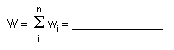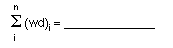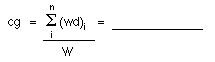PRINT THIS ANSWER SHEET TO FILL OUT AND TURN IN.

NAME_________________________________ CLASS____________________ DATE____________

### Determining Center of Gravity (Level 1) Worksheet

A Boeing 747-400 domestic airplane is soaring the friendly skies (shown below). Use data from the Boeing 747 Wikipedia Website (http://en.wikipedia.org/wiki/Boeing_747) to complete Table 1. Estimate values for data you are unable to locate in a reasonable amount of time. You should be able to find the length of the airplane (for the reference distances requested), the mass of the engine, and the fuel capacity. The distances in Table 1 should be measured from a reference line that starts at the nose (front) of the 747. Use the BACK key to return to this page.

Problem 1 will guide you in the calculation to find the mass of the fuel.

All problems are expressed in metric units.

 Mass (kg) Distance from Reference Line (m) payload d1 engine d2 wings d3 fuselage d4 fuel d5 vertical tail d6 horizontal tail d7
Table 1 : Airplane component masses and distances from reference line

1. What is the fuel capacity of the 747?

Fuel capacity = Volume of fuel = ______________

2. Assuming the density of an average jet fuel is 0.75 g/ml, use the density equation,
density = mass / volume,

to calculate the mass of the fuel. (Hint: You must convert from liters to milliliters.)

Mass of fuel = ______________

Record this value for the mass of the fuel in Table 1.

3. In Table 2, record the weights of the components (parts) listed in Table 1.

(Hint: Remember, F = mag = W. The acceleration due to gravity, ag , is 9.8 m/s2.)

 Mass (kg) Weight (N) payload w1 engine w2 wings w3 fuselage w4 fuel w5 vertical tail w6 horizontal tail w7
Table 2 : Airplane component weights

4. What does n equal?

[Hint: The number n is the number of quantities being added together.]

n = _________

5. What are the values for i?

i = _________________________

6. What is the total weight W of the airplane?7. What is the value of the sum of the component weights times their distances from the reference line (see the equation below)?8. Using your answers from Problems 6 and 7, calculate the center of gravity.9. After a long flight, the amount of fuel left in the tanks is 20% of the initial amount. What is the mass and weight of the fuel that is left? Record your answers in Table 3.
 Mass (kg) Weight (N) Remaining Fuel
Table 3 : Remaining Fuel Data

10. Recalculate the airplane's center of gravity with the reduced fuel weight.11. Did the center of gravity change?

1. If yes, by how many meters did the center of gravity move?

2. If yes, did the center of gravity move toward or away from the nose of the airplane?

3. Do you think the pilot would notice such a change in the center of gravity while flying? Why?